# 考研数据结构与算法（八）查找¶

## 一、基本概念¶

### 1.4 平均查找长度¶

#### 1.4.1 成功平均查找长度¶

$ASL_{成功}=\sum_{i=1}^nP_iC_i$

#### 1.4.2 失败平均查找长度¶

$ASL_{失败}=\sum_{i=1}^mP_iC_i$

## 二、静态查找¶

### 2.1 顺序表（顺序查找）¶

typedef struct{／／查找表的数据结构
ElemType *elem; //元素存储空间基址，建表时按实际长度分配，0 号单元留空
int TableLen;   //表的长度
}SSTable;
int Search_Seq(SSTable ST , ElemType key) {
ST.elem=key; //“哨兵”
for(i = ST.TableLen; ST.elem[i] != key; --i); ／／从后往前找
return i; ／／若表中不存在关键字为 key 的元素，将查找到 i 为 0 时退出 for 循环
}


typedef struct{／／查找表的数据结构
ElemType *elem; //元素存储空间基址，建表时按实际长度分配
int TableLen;   //表的长度
}SSTable;
int Search_Seq(SSTable ST , ElemType key) {
for(i = ST.TableLen - 1; i >= 0; --i); ／／从后往前找
if(ST.elem[i] == key)
return i; ／／表中找到关键字 key
return -1;//表中未找到关键字
}


$ASL_{成功} = \sum_{i=1}^nP_ii = \frac{n+1}{2}$

$ASL_{成功} = \sum_{i=1}^nP_i(n-i+1) = \frac{n+1}{2}$

$ASL_{失败} = \sum_{i=1}^nP_i(n) = \frac{n}{2}$

$ASL_{成功} = \sum_{i=1}^nP_ii = \frac{n+1}{4}$

$ASL_{平均} = ASL_{成功} + ASL_{失败} = \frac{n+1}{4} + \frac{n}{2} = \frac{3n + 1}{4}$

$C_i = \begin{cases} i+1, & \text{if} \ i<n \\ n, & \text{if} \ i=n \end{cases}$

$ASL{失败}=\sum_{i=1}^nP_iC_i = \frac{1}{2n}\sum{i=1}C_i = \frac{1+2+3+……+n+n}{2n} = \frac{n+3}{4}$

### 2.2 有序表（二分查找）¶

$\{7, 10, 13 , 16, 19, 29, 32 , 33 , 37, 41 , 43 \}$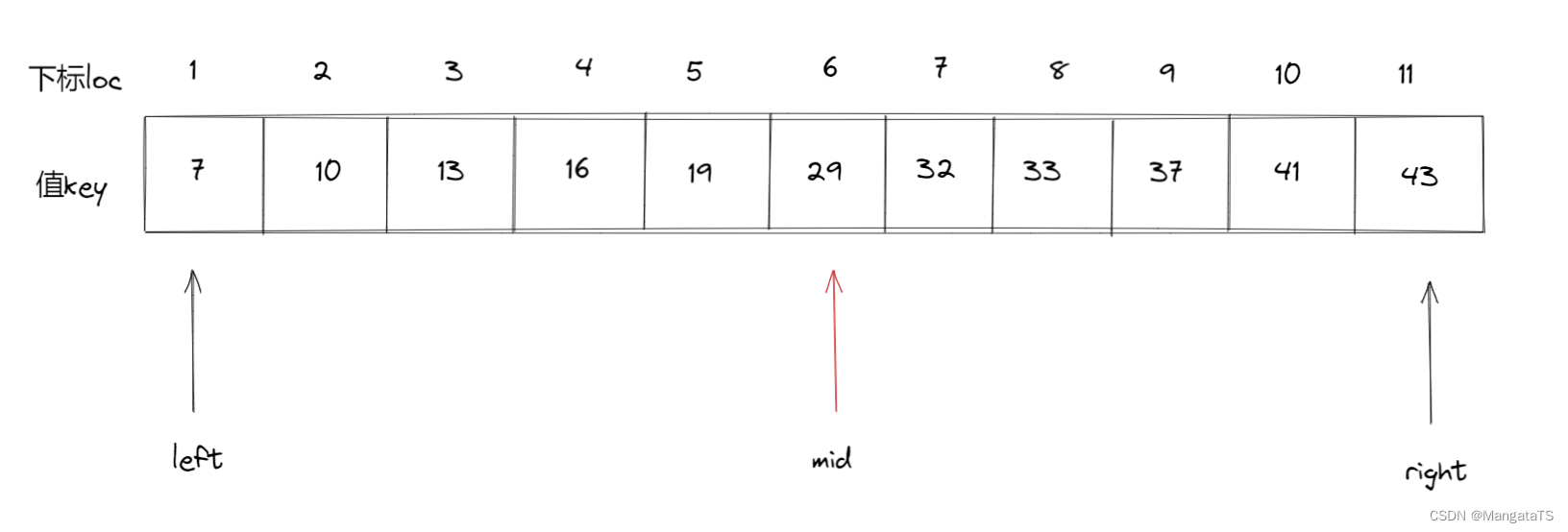• 第一次查找：$$mid = \left \lfloor \frac{\text{left}+\text{right}}{2} \right \rfloor =\left \lfloor \frac{1+11}{2} \right \rfloor = 6$$ 于是我们就将 $$a$$$$32$$ 进行比较，我们发现 $$a < 32$$ 于是我们将 $$left$$ 更新，即 $$left = mid + 1 = 7$$$$right$$ 不变，还是 $$11$$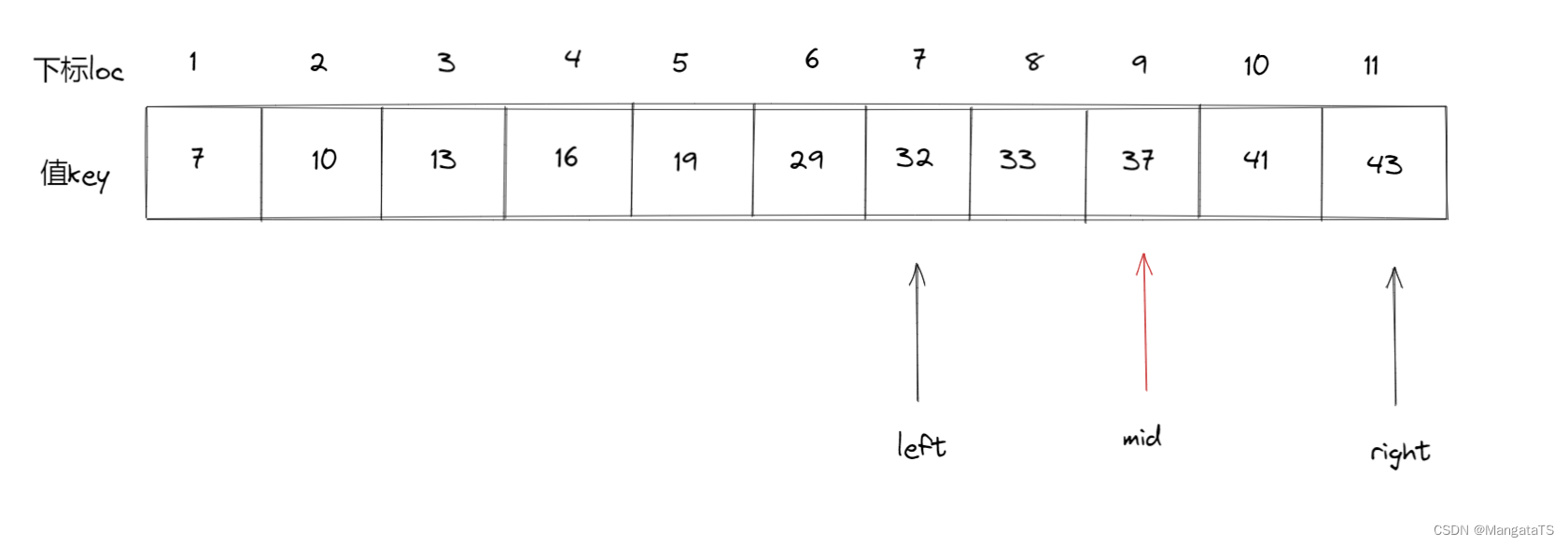• 第二次查找： $$mid = \left \lfloor \frac{\text{left}+\text{right}}{2} \right \rfloor =\left \lfloor \frac{7+11}{2} \right \rfloor = 9$$ 于是我们将 $$a$$$$32$$ 进行比较，我们发现 $$a > 32$$ 于是我们将 $$right$$ 更新，即 $$right = mid - 1 = 8$$$$left$$ 不变，还是 $$7$$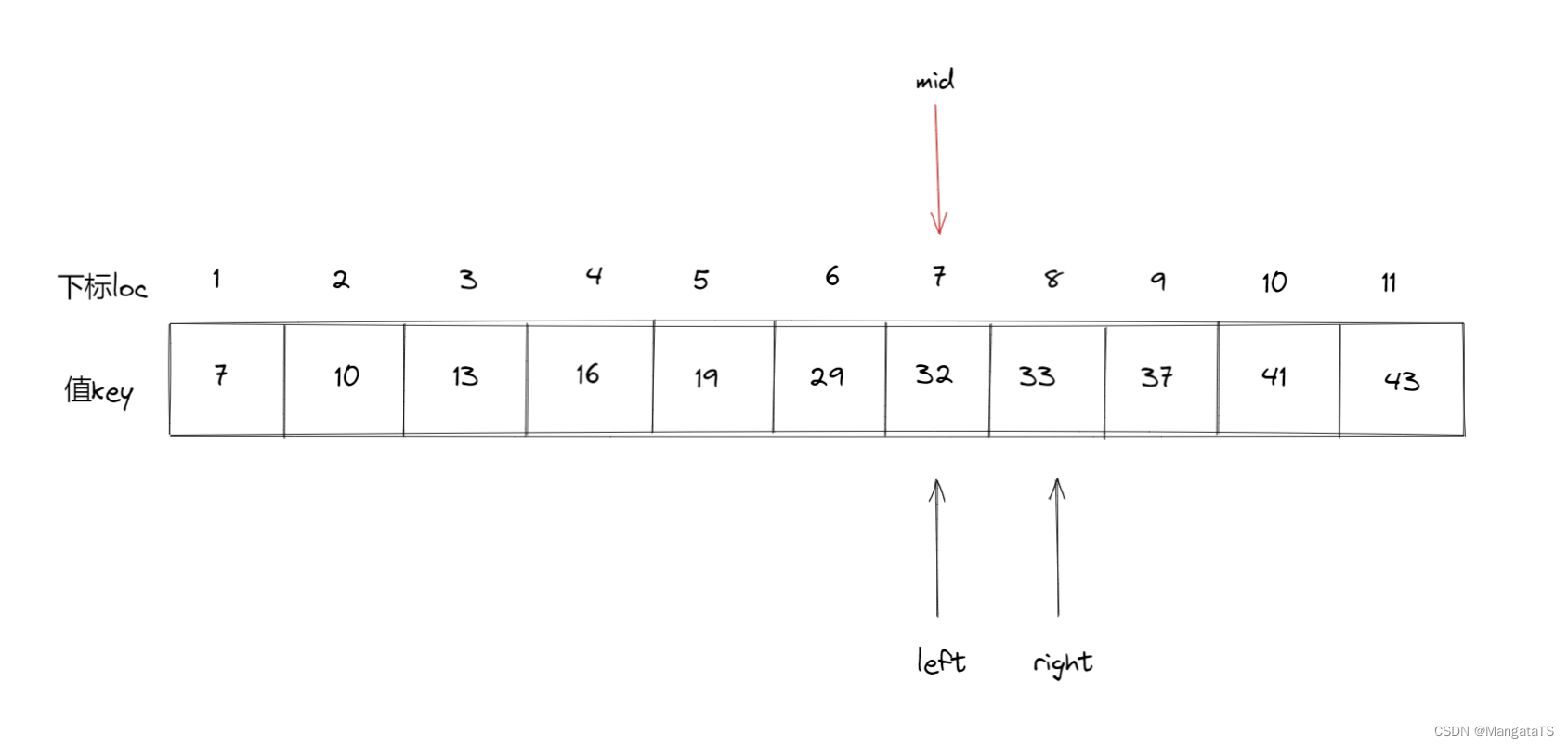• 第三次查找： $$mid = \left \lfloor \frac{\text{left}+\text{right}}{2} \right \rfloor =\left \lfloor \frac{7+8}{2} \right \rfloor = 7$$ 于是我们将 $$a$$$$32$$ 进行比较，我们发现 $$a = 32$$ 此时我们就找到了这个元素，那么我们将当前位置返回，至此查找完成~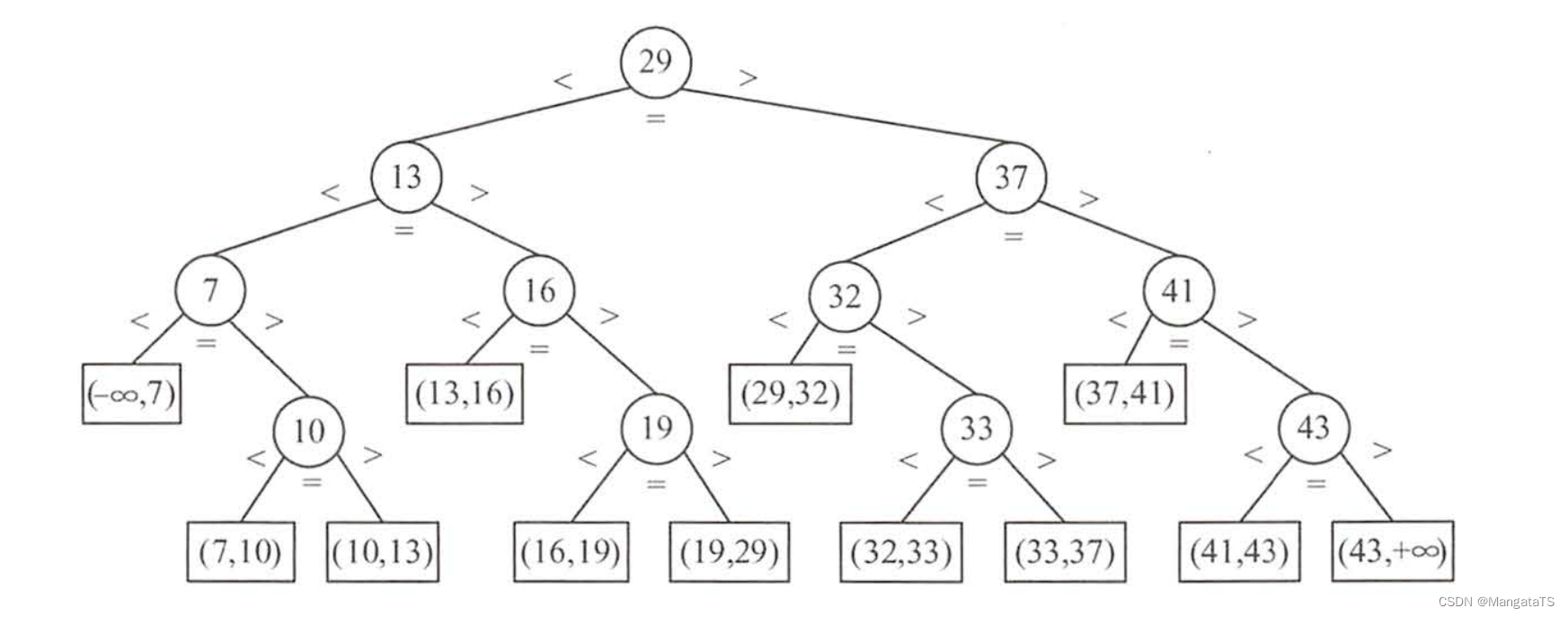$ASL_{成功} = \frac{1}{n}\sum_{i=1}^nL_i=\frac{1\times 1 + 2\times 2 + 3\times 3 + …… + h \times 2^{h-1}}{n} = \frac{n+1}{n}log_2(n+1)-1 ≈ log_2(n+1)-1$

$ASL_{失败} = \frac{1}{m}\sum_{i=1}^nL_i = \frac{1\times D_1 + 2\times D_2 + 3\times D_3 + …… + h \times D_h}{m}$

### 2.4 索引表（分块查找）¶

#### 2.4.2 查找步骤¶

• ①第一步是在索引表中确定待查记录所在的块
• ②再块内顺序查找

#### 2.4.3 举例¶

• 按照关键字我们分为四个块： $$24、54、78、88$$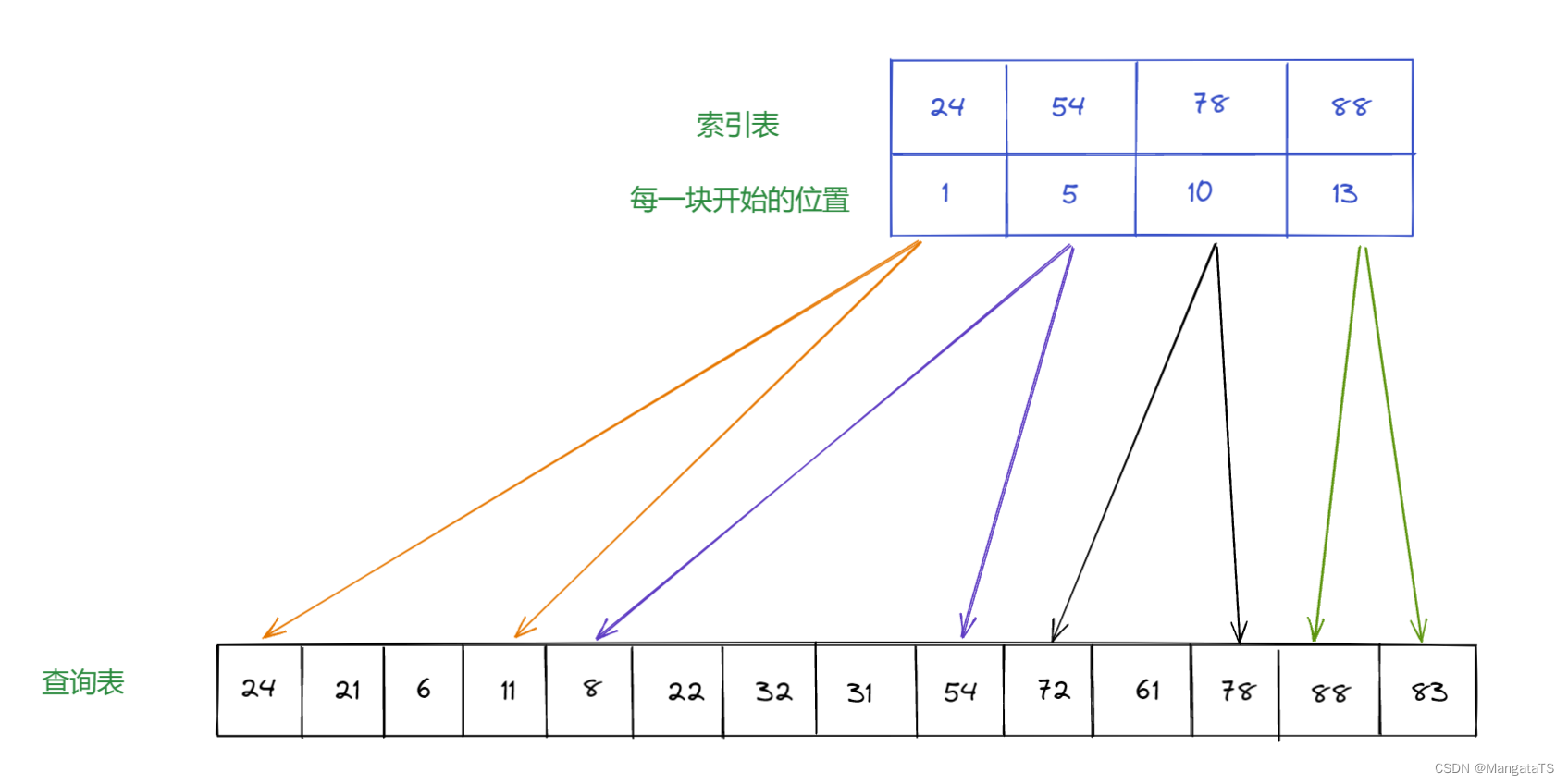ps：这里的索引表构造可以有多种情况

• 若是我们想要找 $$61$$ 那么我们就需要先去在索引表中顺序查找（或是二分查找）我们所需的元素可能出现在哪一块，我们发现 $$61$$ 大于第二块表的最大值，并且小于第三块表的最大值，那么 $$61$$可能存在 第三块表中
• 于是我们从下标 $$10$$ 开始查找一直到 $$12$$ （即这一块的范围），因为表内可能是乱序的，于是我们使用顺序查找的方法，然后找到了 $$61$$ 的下标为 $$11$$ 这就是一个查找的过程

$ASL_{成功} = L_i + L_s$

$ASL_{成功} = L_i + L_s = \frac{b+1}{2} + \frac{s+1}{2} = \frac{s^2+2s+n}{2s}$

ps:

$∵sb=n \\ ∴ b = \frac{n}{s}$

$ASL = L_i + L_s = \left \lceil log_2(b+1) \right \rceil + \frac{s+1}{2}$

## 三、动态查找¶

### 3.5 键树（Trie树）¶

Trie (字典)树详解：https://acmer.blog.csdn.net/article/details/120058928